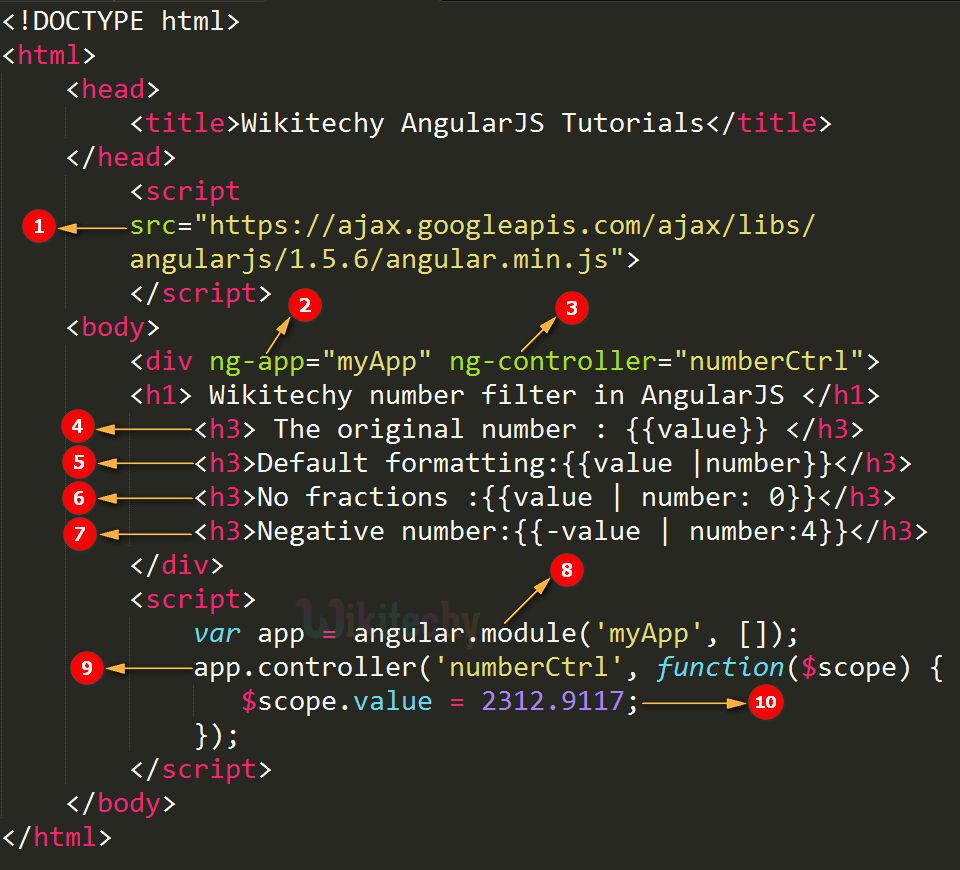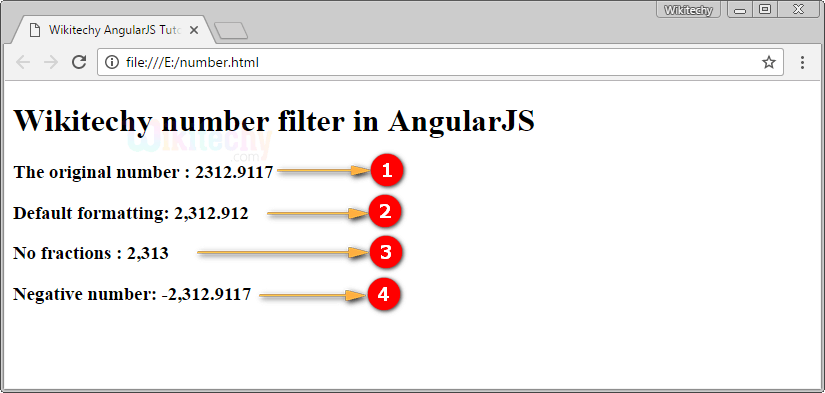# AngularJS Number

• The number filter is used to format a number as a string or text.
• The input value must be a number, otherwise it will just be return.
• The fractionSize value is used to define the number of decimal.

## Parameter value for number filter in AngularJS:

Value Type Description
fractionSize number
string
The fractionSize is an optional value. if The value must be a number. It is used to specifying the number of decimal. The default value of fractionSize is 3.

## number Filter in AngularJS:

• The number filter used to displays a different number format in our AngularJS application.

## Data:

• The data has been defined for our AngularJS Application.

## Logic:

• Controller logic for the AngularJS Application.

## HTML:

• Viewable HTML contents in AngularJS Application.

## Code Explanation for number filter in AngularJS:1. AngularJS is distributed as a JavaScript file, and can be added to a HTML page with a <script> tag.
2. The AngularJS application is defined by ng-app="myApp". The application runs inside the <div> tag. It’s also used to define a <div> tag as a root element.
3. The ng-controller=”numberCtrl” is an AngularJS directive. It is used to define a controller name as “numberCtrl”.
4. {{value}} is used to bind a input value.
5. The {{value | number}} is used to bind a default number format. Here the original input value is displayed with the comma and three decimal point, for example (2,312.912)
6. The {{value | number: 0}} is used to bind a no fraction number format. Here the input value displayed without decimal, for example (2,313).
7. The {{-value | number: 4}} is used to bind a negative number format. Here the input number is displayed with negative symbol and four decimal point, for example (-2,312.9117)
8. The angular.module function is used to create a Module. We have passed an empty array to it.
9. Here we have declared a controller numberCtrl module using apps.controller() function. The value of the controller modules is stored in scope object. In AngularJS, \$scope is passed as first argument to apps.controller during its constructor definition.
10. Here we have set the value of \$scope.value=2,312.9117; which are to be used to display the number format values in the HTML <div> element.

## Sample Output for number filter in AngularJS:1. The output displays an original input value by using {{value}}.
2. The output displays a default number formatting by using a number filter.
3. The output displays a no fraction number format by using a number filter.
4. The output displays a negative number format by using a number filter.